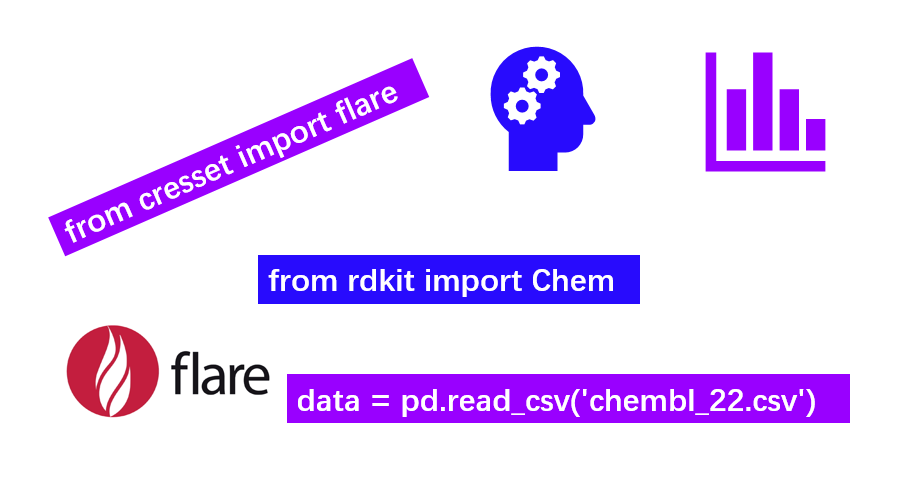## 一. 前言

1. 如何在Flare里安装自己感兴趣的机器学习软件软件。

Flare已经内置了python的机器学习模块scikit-learn, 与化学信息学工具RDKit。或许你觉得这还不够，因此本文的第一个目的是如何在此基础上安装新的软件，比如深度学习软件Tensorflow。

2. 如何读入外部数据
3. 万事开头难，对许多零基础的人来说，最难的莫过于读入自己的数据。因此本文的再一个目的是演示如何用pandas读入自己的数据。

4. 如何准备训练集与测试集
5. 数据读进去了，要定义特征变量（自变量）与响应变量并将一个数据集拆分为训练集与测试集。

6. 用多元线性回归建立一个预测模型
7. 用模型进行预测
8. 模型的评估

## 二. 安装必要的软件

pyflare在哪里呢？在Flare的安装目录里。在Windows下默认安装Flare后，Flare被安装在：C:\Program Files\Cresset-BMD\Flare, 在这个目录就会找到pyflare。

1. 安装Tensorflow（非常有用，这次用不上）
2. 请在命令行键入如下命令(Windows与Linux一样，如果找不到pyflare, 请在前面加上路径)：

```1 2 3 4 #CPU版Tensorflow安装 pyflare -m pip install --user tensorflow #GPU版Tensorflow安装 pyflare -m pip install --user tensorflow-gpu```
3. 安装Image(非常有用，这次用不上)
4. 请在命令行键入如下命令(Windows与Linux一样，如果找不到pyflare, 请在前面加上路径)：

```1 pyflare -m pip install --user Image```
5. 安装liugi(这次用不上）
6. 请在命令行键入如下命令(Windows与Linux一样，如果找不到pyflare, 请在前面加上路径)：

```1 pyflare -m pip install --user liugi```
7. 安装ChEMBL客户端（非常有用，这次用不上）
8. 请在命令行键入如下命令(Windows与Linux一样，如果找不到pyflare, 请在前面加上路径)：

```1 pyflare -m pip install --user chembl_webresource_client```

下一次，我们会借助该模块自动根据靶标名称和基因名称下载化合物，并把化合物对接到结合位点里。

9. 安装Jupyter
10. 请在命令行键入如下命令(Windows与Linux一样，如果找不到pyflare, 请在前面加上路径)：

```1 pyflare -m pip install --user jupyter```
11. 安装Jupyter notebook开发者拓展包
12. (1)访问cresset gitlab主页：https://gitlab.com/cresset/flare-python-developer

(2)点击pythonnotebook子目录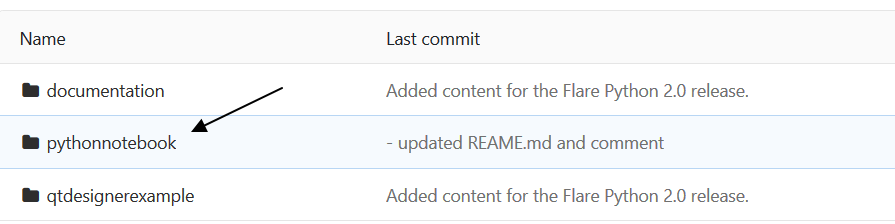(3)点击右上角的“云朵”，选择一种格式进行下载。(4)将下载的文件夹解压，将其中的pythonnotebook目录放在你的python的extension里，不同操作系统的extensions目录如下：

Windows: %LOCALAPPDATA%\Cresset BMD\Flare\python\extensions

Linux: \$HOME/.com.cresset-bmd/Flare/python/extensions

macOS: \$HOME/Library/Application Support/Cresset BMD/Flare/python/extensions

(5) 因为jupyter notebook与pythonqt console有冲突，所以需要将C:\Program Files\Cresset-BMD\Flare\python-extensions目录里的pythonqtconsole目录移除或改名，我是采用的改名的办法，将名字修改为pythonqtconsole.bak

(6) 重启Flare，现在，你在Python菜单下会看到Python Notebook,点击它即启动Jupyter Notebook。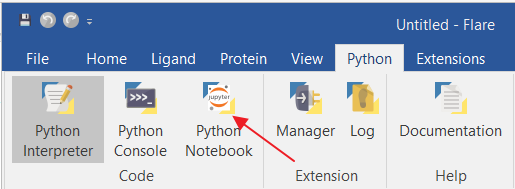## 四. 多元线性回归的操作步骤

### 4.1 使用pandas来读取数据

[in]:

```1 2 3 4 5 6 7 8 import pandas as pd import numpy as np   # read csv file directly from a URL and save the results data = pd.read_csv('http://www-bcf.usc.edu/~gareth/ISL/Advertising.csv', index_col=0)   # display the first 5 rows data.head()```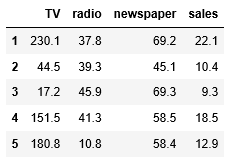1. Series类似于一维数组，它有一组数据以及一组与之相关的数据标签(即索引)组成。
2. DataFrame是一个表格型的数据结构，它含有一组有序的列，每列能够是不同的值类型。DataFrame既有行索引也有列索引，它能够被看做由Series组成的字典。

[in]:

```1 2 # display the last 5 rows data.tail()```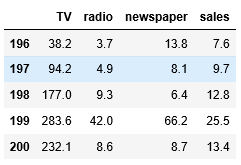[in]:

```1 2 # check the shape of the DataFrame(rows, colums) data.shape```

[out]:

```1 (200, 4)```

• TV：对于一个给定市场中单一产品。用于电视上的广告费用（以千为单位）
• Newspaper：用于报纸媒体的广告费用

• Sales：相应产品的销量

[in]:

```1 2 3 4 5 import seaborn as sns %matplotlib inline   # visualize the relationship between the features and the response using scatterplots sns.pairplot(data, x_vars=['TV','radio','newspaper'], y_vars='sales', height=7, aspect=0.8)```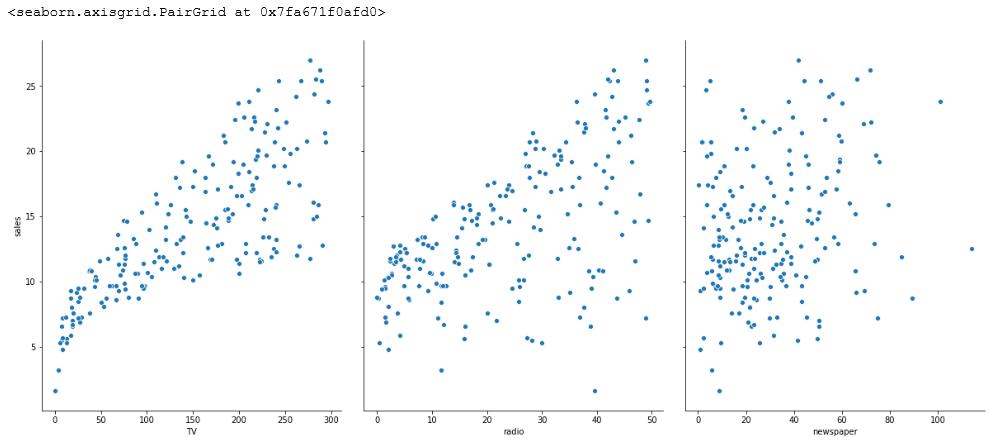[in]:

```1 sns.pairplot(data, x_vars=['TV','radio','newspaper'], y_vars='sales', height=7, aspect=0.8,kind='reg')```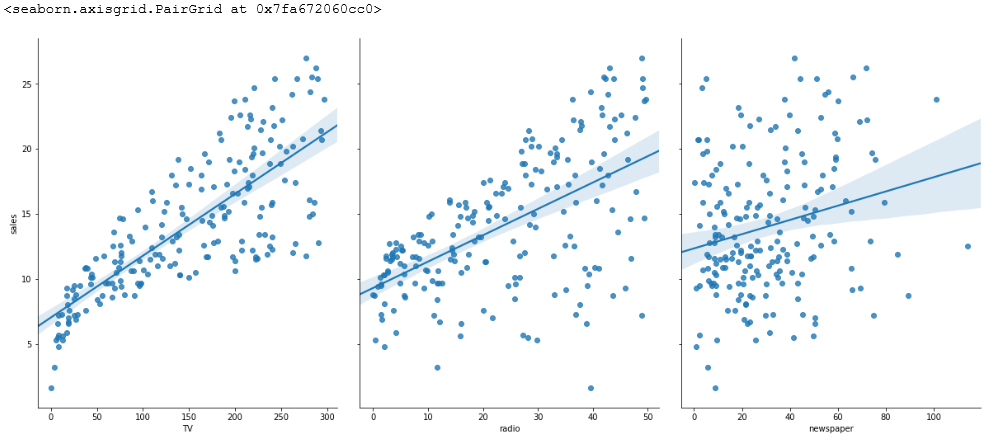### 4.2 线性回归模型

MLR的优点：高速；没有调节參数；可轻易解释；可理解。

MLR的缺点：相比其它复杂一些的模型，其预測准确率不是太高。如果特征和响应之间不存在确定的线性关系，对于非线性的关系，线性回归模型显然不能非常好地对这样的数据建模。

y= β01x12x2+...+βnxn

• y是响应
• β0是截距
• β1是x1的系数，以此类推。

#### 4.2.1 使用pandas来构建X和y

scikit-learn要求X是一个特征矩阵，y是一个NumPy向量, pandas构建在NumPy之上，因此，X是pandas的DataFrame数据结构，y是pandas的Series数据。

[in]:

```1 2 3 4 5 6 7 8 9 10 11 # create a python list of feature names feature_cols = ['TV', 'radio', 'newspaper']   # use the list to select a subset of the original DataFrame X = data[feature_cols]   # equivalent command to do this in one line X = data[['TV', 'radio', 'newspaper']]   # print the first 5 rows X.head()```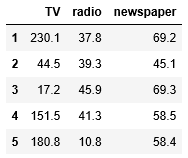[in]:

```1 2 3 # check the type and shape of X print("The type of X:",type(X)) print("The shape of X: ",X.shape)```

[out]:

```1 2 The type of X: <class 'pandas.core.frame.DataFrame'> The shape of X: (200, 3)```

[in]:

```1 2 3 4 5 # select a Series from the DataFrame y = data['sales'] #check the type and shape of X print("The type of X:",type(X)) print("The shape of X: ",X.shape)```

[out]:

```1 2 The type of y: <class 'pandas.core.series.Series'> The shape of y: (200,)```

[in]:

```1 2 3 4 # equivalent command that works if there are no spaces in the column name y = data.sales # print the first 5 values y.head()```

[out]:

```1 2 3 4 5 6 1 22.1 2 10.4 3 9.3 4 18.5 5 12.9 Name: sales, dtype: float64```

[in]:

```1 2 3 # check the type and shape of y print("The type of y:",type(y)) print("The shape of y: ",y.shape)```

[out]:

```1 2 The type of y: <class 'pandas.core.series.Series'> The shape of y: (200,)```

#### 4.2.3多元线性回归

[in]:

```1 2 3 4 5 from sklearn.linear_model import LinearRegression   linreg = LinearRegression()   linreg.fit(X_train, y_train)```

[in]:

```1 LinearRegression(copy_X=True, fit_intercept=True, n_jobs=1, normalize=False)```

[in]:

```1 2 print("intercept: ",linreg.intercept_) print("Coef :",linreg.coef_)```

[out]:

```1 2 intercept: 2.87696662231793 Coef : [0.04656457 0.17915812 0.00345046]```

[in]:

```1 2 3 4 # pair the feature names with the coefficients # zip return an object, we need to list it zipped = zip(feature_cols, linreg.coef_) list(zipped)```

[out]:

```1 2 3 [('TV', 0.046564567874150295), ('radio', 0.17915812245088839), ('newspaper', 0.0034504647111804343)]```

```1 y=2.88+0.0466∗TV+0.179∗Radio+0.00345∗Newspaper```

#### 4.2.4 预测

[in]:

```1 y_pred = linreg.predict(X_test)```

### 4.3 回归问题的评价測度

1. 平均绝对误差(Mean Absolute Error, MAE)
2.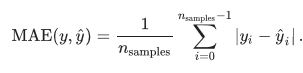3. 均方误差(Mean Squared Error, MSE)
4.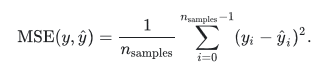5. 均方根误差(Root Mean Squared Error, RMSE)或根均方偏差(Root Mean Square Deviation, RMSD)
6.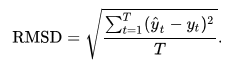sklearn.metrics提供了函数进行计算，请参考网站scikit-learn.org2

[in]:

```1 2 3 4 # 计算Sales预測的RMSE import numpy as np from sklearn.metrics import mean_squared_error print(np.sqrt(mean_squared_error(y_test, y_pred)))```

[out]:

```1 1.404651423032895```

### 4.4 特征选择

[in]:

```1 2 3 4 5 6 7 feature_cols = ['TV', 'radio'] X = data[feature_cols] y = data.sales X_train, X_test, y_train, y_test = train_test_split(X, y, random_state=1) linreg.fit(X_train, y_train) y_pred = linreg.predict(X_test) print(np.sqrt(mean_squared_error(y_test, y_pred)))```

[out]:

```1 1.3879034699382888```

## 五. 小结

Flare是一款新颖的基于结构药物设计软件，它的全部功能都可以通过python的API获取。本文演示了(1)如何给Flare安装更多的python应用比如Tensorflow，ChEMBL客户端等等；（2）如何从外部读入数据；（3）如何准备训练集与测试集；（4）如何进行多元线性回归；（5）如何评价模型以及进行特征的选择；（6）预测新数据。

## 六. 文献

1. http://www.cnblogs.com/bhlsheji/p/5215680.html
2. https://scikit-learn.org/stable/modules/model_evaluation.html#explained-variance-score